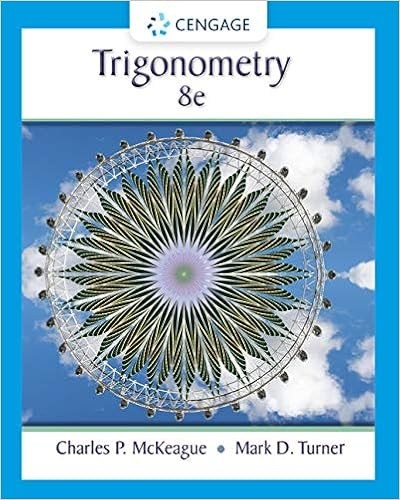# U1L1_Exercises - Selected Exercises Distance and Midpoint...

• Notes
• cherelle6655
• 5
• 100% (1) 1 out of 1 people found this document helpful

This preview shows page 1 - 5 out of 5 pages.

##### We have textbook solutions for you!
The document you are viewing contains questions related to this textbook.The document you are viewing contains questions related to this textbook.
Chapter 1 / Exercise 60
Trigonometry
McKeague/TurnerExpert Verified
Selected Exercises Distance and Midpoint Graphs of Equations – Symmetry in Graphs 5.Select all of the following graphs which represent yas a function of x. ab cd e f
##### We have textbook solutions for you!
The document you are viewing contains questions related to this textbook.The document you are viewing contains questions related to this textbook.
Chapter 1 / Exercise 60
Trigonometry
McKeague/TurnerExpert Verified
6.Select all of the following graphs which represent yas a function of x. abcde f13.Select all of the following graphs which are one-to-one functions. a.b.c.d.e. f.
14.Select all of the following graphs which are one-to-one functions. abcde f Given each function ( )f xgraphed, evaluate (1)fand (3)f15.16.
Section 5.1 (Equations of Circles) 3.Write an equation of the circle centered at (8 , -10) with radius 8. 5.Write an equation of the circle centered at (7, -2) that passes through (-10, 0). 6.Write an equation of the circle centered at (3, -7) that passes through (15, 13). 8. Write an equation for a circle where the points (-3, 3) and (5, 7) lie along a diameter. 10. Sketch a graph of ()()221216xy++=11. Find the yintercept(s) of the circle with center (2, 3) with radius 3. 13. At what point in the first quadrant does the line with equation .
•••